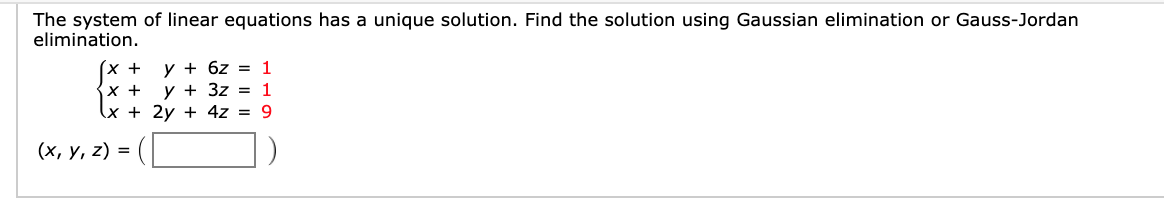# The system of linear equations has a unique solution. Find the solution using Gaussian elimination or Gauss-Jordan elimination. (x + x + x + 2y + 4z = 9 y + 6z = 1 y + 3z = 1 (х, у, 2) %3D

Questionhelp_outlineImage TranscriptioncloseThe system of linear equations has a unique solution. Find the solution using Gaussian elimination or Gauss-Jordan elimination. (x + x + x + 2y + 4z = 9 y + 6z = 1 y + 3z = 1 (х, у, 2) %3D fullscreen

### Want to see this answer and more?

Experts are waiting 24/7 to provide step-by-step solutions in as fast as 30 minutes!*

*Response times may vary by subject and question complexity. Median response time is 34 minutes for paid subscribers and may be longer for promotional offers.
Tagged in
MathCalculus

### Other